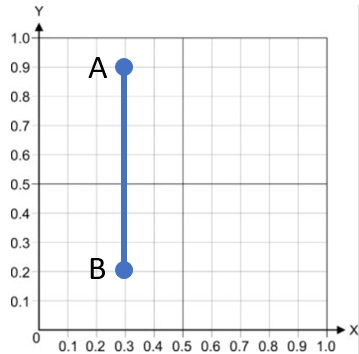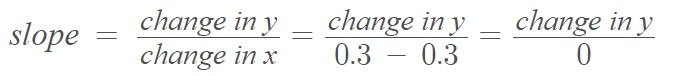# Infinite Slope Example

## Infinite Slope Example: Calculus

Vertical lines have an infinite slope. For example, the following graph has an infinite slope:When you plot a vertical line on a Cartesian plane, an infinite slope runs parallel to the y-axis.

It doesn’t matter which two points you choose on the graph to calculate the slope, the x-value stays the same. For the above graph, that is x = 0.3. If we try and plug that into the slope formula, we get division by 0, which is undefined:By convention, we usually call the slopes of vertical lines “undefined slopes“, because of the division by zero problem. In addition, it isn’t possible for a perfectly vertical line to have any kind of “slope” at all so it seems to make sense to called them undefined. However, it’s fairly common for an undefined slope to be called infinite slopes or “no slope” , which could be confused with the zero slope of a horizontal line. As we don’t really know whether these slopes tend towards positive or negative infinity, the term undefined slope is usually preferred. However, for most intents and purposes, the subtle difference between the terms is usually not important.

## Infinite Slope Analysis

In geology, the concept of an infinite slope is different from that of a simple vertical line. It is a slope that extends for a “relatively long distance” . Infinite slope analysis, important for forecasting landslides and other geological movements, has several assumptions: the failing mass is of infinite lateral extent, of uniform thickness, and the failure surface is perfectly planer. It’s also assumed that the normal and tangential forces on the upslope
side and downslope side of an element of the slope are equal .

## References

 Math 45 SSM 2/e 3.3 Slope p.1.
 Civil Engineering Bible. Infinite Slope Analysis. Retrieved November 28, 2021 from: https://civilengineeringbible.com/subtopics.php?i=68
 Geomorphic Processes 15-GEO-504.

CITE THIS AS:
Stephanie Glen. "Infinite Slope Example" From StatisticsHowTo.com: Elementary Statistics for the rest of us! https://www.statisticshowto.com/infinite-slope-example/